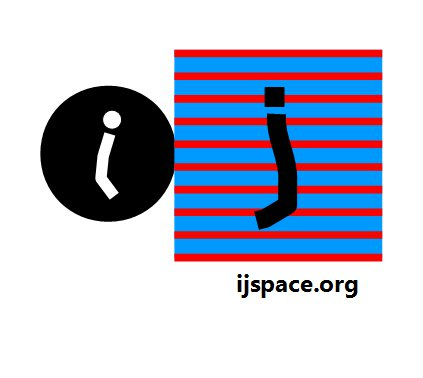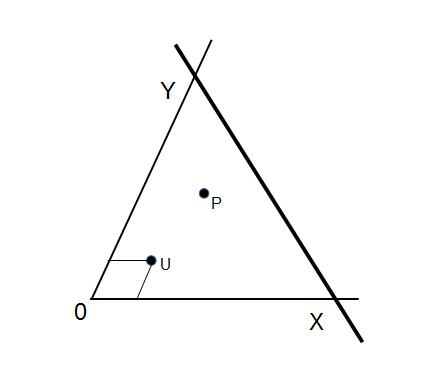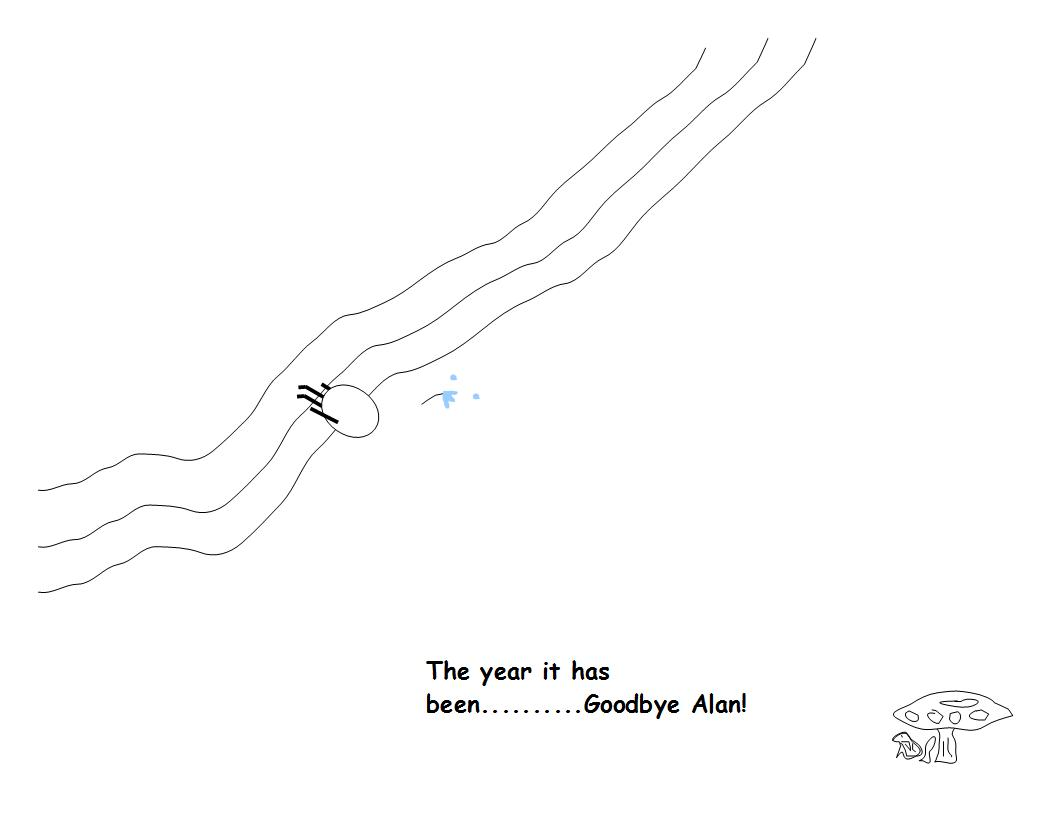# Home

 In a Nutshell-2015 26th December 2015 In the year 2015 we examined some very interesting ideas.  During our investigations we looked into the rationale behind the arithmetic operations.  We found out that in a true discrete measurement space or j-space where each point was unique, the basic arithmetic operations, such as addition, subtraction, multiplication, and division were not possible.  We had already dispensed with the notion of time, unless we developed description in (t, x, y, z, m, q) metric corresponding to the macroscopic observer ObsM situated in Λ∞ plane.  Then what type of knowledge could we possibly gain without basic arithmetic operations and the notion of time?     There are some truly extraordinary concepts which transcend any branch of science.  ZFC axioms are one of them.  We realized that all the axioms would require an assumption of some sort of symmetry for them to be valid in j-space, except for the Axiom of Foundation.  The Axiom of Foundation stated that "Every non-empty set X contains an ∈-minimal element, that is, an element such that no element of X belongs to it.", i.e. ¬∈    =    ∈ . The discrete j-space required that negation of a measurement must also corresponded to a measurement.  Further we knew that a true null-set could not exist in j-space as it could not be precisely measured as null.  Thus we had to consider the possibility that the negation of a measurement was equal to the measurement itself due to the lack of resolution.  This state must occur just beyond the Observer's measurement threshold.  It could be considered an essential characteristic of an observer whose capacity to measure a source, is infinitesimally small.     If we put our predicament in front of Obsi, even he (or she) would agree that such state should exist in j-space for the macroscopic observer.  This rather fascinating and quite unexpected development enabled us to differentiate between Majorana and Dirac descriptions, with Majorana states being the states with information content higher than Dirac states.     We next introduced arguably one of the most original ideas, the incredible Anharmonic Coordinates by Hamilton.  It allowed us to understand the significance of the z-axis used to measure spin in quantum-mechanics.  The Anharmonic Coordinates (Unit-point) were very different than Barycentric Calculus (Center-of-Gravity), even though they originated from the ideas behind Barycentric Calculus developed first by Möbius.     Associated with Anharmonic Coordinates was the elegant geometrical construction ΔOXY,  and the concept of the unit-point U.  The beauty lied in drawing the line XY and then defining the unit-point U(111) in terms of the vertices O(001), X(100), and Y(010).  It should be noted that the conventional origin (000) was represented as O(001), thus giving the origin, weightage equal to the other two vertices.  We were able to construct a geometrical net of measurements which consisted of only the rational points.  The points not lying on the net would be irrational points.This geometrical net was used to describe an arbitrary point P with respect to the unit-point U(111) instead of origin O(001).  The translation of the reference point from O(001) to U(111) was important, as it allowed us to visualize finiteness inherent in any description developed in anharmonic coordinates.     The anharmonic coordinates could be used for any two-state system with interaction between pure-states being accounted for.  And we were not required to use either four-vector description or tensors, in a two-state system to explain finiteness implicit in the unit-point U(111).  The the physical quantities (eg. m or q), corresponding to "finiteness" in Λ∞ plane, would be different for observers with different capacities following different symmetries.   An important point which needed to be emphasized here, was that OX and OY axes were not orthogonal in Anharmonic Coordinates.  Therefore the measurements made along OX and OY axes were not necessarily independent of each other.  The duality we were so accustomed to in macroscopic word or Λ∞ plane, would be true only if we assumed that the axes OX and OY were orthogonal.  Only then we could successfully apply Complex Algebra and visualize electron-photon structure (q = 3 state or lower information state).  This was exactly what we did when we used Pauli's Spin Matrices along with anharmonic coordinates to develop unit-point sphere.     The internal symmetry or 4π-symmetry was described by applying the Pauli's Spin Matrices to ΔOXY.  The concepts of the unit-point sphere SU and the Λ∞-plane, location of the macroscopic observer (AKU: All Knowing Us), were established.  We also discussed the existence of the shell-structure and the central-force nature of the problem as one of the consequences of application of the spin matrices to the anharmonic coordinates.         One of the outcome was that we would have to associate the quantum nature to the interactions or measurements rather than entities such as electron or photon, which themselves were measurements of a macroscopic observer.  Then how would we visualize the wave-particle duality?  Well, we must establish conditions under which a wave-equation could be used to describe a measurement.     The angle-XOY, represented the scale on which the information content of a state as measured by an observer could be calibrated.  Thus we could compare the measurement capacities of different observers.  Smaller the angle, higher the information content, higher the resource requirement for the measurement and higher the observer capacity.What was the advantage? Let us say if we could raise the observer's capacity just a little bit beyond the existing  (q = 3) or (e-ν)  structure within an infinitesimal confinement under controlled conditions, to (q = 3-ε)  or equivalently (e'-ν') structure.  In such case we could possibly alter the nature of interaction just enough to reduce say friction for argument sake, within the confinement.  Smaller the angle-XOY, smaller should be the effect of macroscopic forces (so familiar to us) in the confinement.  But first we need to formulate the capacity of the (q = 3)-observer in terms of the physical constants we could measure.  A formidable task! But if it could be done then maybe Beijing could breathe again.     To understand the conventional symmetries we introduced an apparatus which is equivalent to a mirror in Λ∞-plane.  Using this apparatus we were able to describe chiral-symmetry and nature-preferred state.  We discussed the exclusion principle as applicable to the discrete measurement space.  It was important to note that the spin matrices form their own inverses, a condition which was not possible in true j-space without the existence of a symmetry.     We had mentioned earlier, the difficulty in visualizing continuity in j-space, even after the VT-symmetry was used to define the origin.  We were able to somewhat resolve this issue by using zero-trace matrices and convex structures.  These matrices described physical entities with no internal structures.  The spin-matrices were examples of zero-trace matrices.  We are still working on developing the concepts related to continuity in  Λ∞-plane.  We can not develop a (t, x, y, z, m, q) description without a clear understanding of continuity.  So far this has been the most difficult task, although the concepts in topology have been very useful.     Currently our immediate objective is to look into the properties of a system described using Anharmonic Coordinates, in more detail.  We will then analyze the ideas behind Noether's theorem and conservation, EPR paradox, and Copenhagen interpretation of the quantum-mechanics.     We are yet to evaluate comprehensively the relationship ∫|Ψ |2 dX = 1.  Before that we will have to explain the relationship between an infinite source and the observer measuring it.  It will also be nice to be able to explain the physical significance of the Planck's constant and Boltzmann's constant in context to j-space and the macroscopic observer.     A very important consideration has been the existence in 3-D.  Our explanation has been less than satisfactory in this matter.  The possibility is that Zörk, our friend in (q = 2)-space, is also restricted to 3-D space, although an observer situated at Λ∞-plane in (q = 3)-space, may want to explain (q = 2)-space using higher dimensions.  We will attempt another argument to explain 3-D constraint based on pure geometry.  And we are yet to complete our discussion on Knot theory, the way we feel it should be correlated to a physical description.      Whatever may come as we move into 2016 we can always ask a simple question, Can we connect two points with a straight line? Previous Blogs:   "The purpose of geometry is to draw us away from the sensible and the perishable to the intelligible and eternal." - Plutarch Ref.: The art of the intelligible: An Elementary Survey of Mathematics in its Conceptual Development by John L. BellBest viewed with Internet Explorer